# VBA如何计算健保应负担金额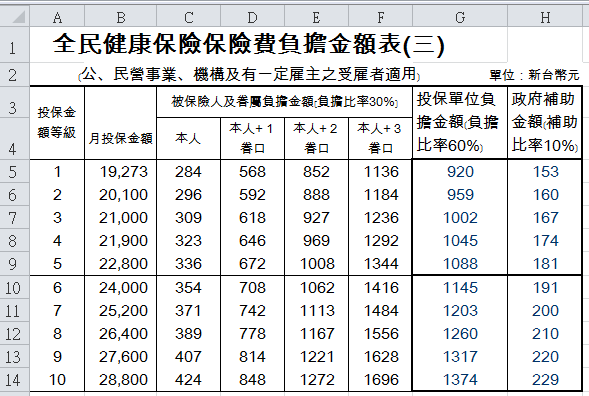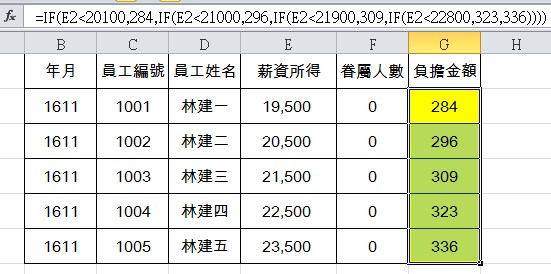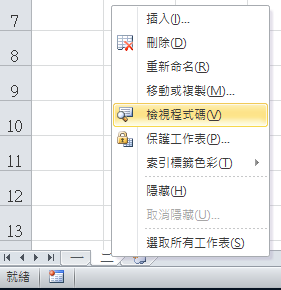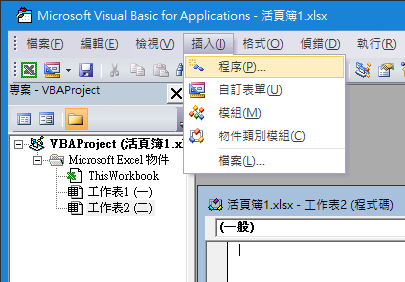Public Sub 健保级距计算（）

For i = 2 To 6 If Cells(i, “D”) < 20100 Then Cells(i, “G”) = 284

If Cells(i, “D”) >= 20100 And Cells(i, “D”) < 21000 Then Cells(i, “G”) = 296

If Cells(i, “D”) >= 21000 And Cells(i, “D”) < 21900 Then Cells(i, “G”) = 309

If Cells(i, “D”) >= 21900 And Cells(i, “D”) < 22800 Then Cells(i, “G”) = 323

If Cells(i, “D”) >= 22800 And Cells(i, “D”) < 24000 Then Cells(i, “G”) = 336

Next

End Sub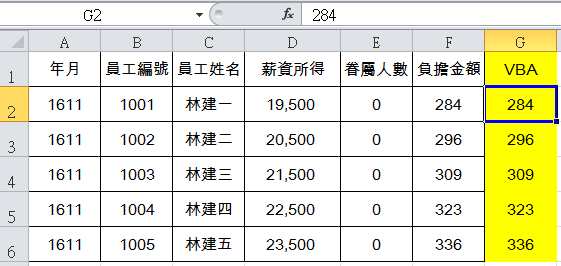Visual Basic for Applications，听起来艰涩，似乎专属于资工系资管系的工程师领域，然而以这篇文章的VBA程序来看，即便不多作说明，应该也能看得懂这几段程序在「讲」什么，这是BASIC语言自1960s年代延续下来的特色，接近自然语言，因此容易理解、也便于学习。套句周爷老电影台词：「只要有心，人人都可以是食神，」在Excel世界里，只要有心，人人都可以让VBA飞！VBA